0  447250  447258  447264  447268  447274  447276  447280  447286  447288  447294  447300  447304  447306  447310  447316  447318  447324  447328  447330  447334  447336  447340  447342  447344  447345  447346  447348  447348

16.(本小题满分8分)设f(x)是一次函数，f(8)=15,且f(2),f(5),f(4)成等比数列，求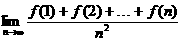.

f (2), f (5), f (4)成等比数列,

∴(5k+b)2=(2k+b)(4k+b). 　　　2分

b=15-8k代入，

f(x)=4x-17.　　　 4分

f(1)+f(2)+…+f(n)

=(4×1-17)+(4×2-17)+…+(4×n-17)

=4×(1+2+…+n)-17n

=4·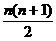-17n=2n2-15n.　　 6分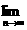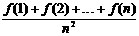=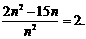8分

15.(本小题满分8分)平面内有n个圆,其中每两个圆都相交于两点,并且每三个圆都不相交于同一点,求证:n个圆把平面分成f(n)=n2-n+2个部分.

(2)假设n=k(k∈N*)时,命题成立,即满足题设条件的k个圆把平面分成f(k)=k2-k+2个部分.2分

14.已知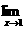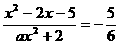,则a的值为　　　　　 .

13.★设函数x=0处连续,则实数a的值为　　　　　 .12.(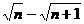)=　　　　　　　　　 .(n-n+1)=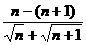=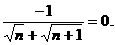11.用数学归纳法证明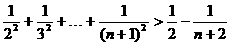,假设n=k时,不等式成立,则当n=k+1时,应推证的目标不等式是　　　　　　　 .

10.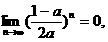a的取值范围是(　　 )

A.a=1　　　　　　　　　　　　 B.a＜-1或a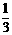C.-1＜aD.a＜-a＞1a＜-1或a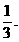.

9.★用数学归纳法证明命题“n3+(n+1)3+(n+2)3(n∈N)能被9整除”,要利用归纳假设证n=k+1时的情况,只需展开(　　)

A.(k+3)3　　　　　B.(k+2)3

C.(k+1)3　　　　　　　　　D.(k+1)3+(k+2)3

8.★欲用数学归纳法证明对于足够大的自然数n,总有2n>n3,n0为验证的第一个值,则(　　 )

A.n0=1

B.n0为大于1小于10的某个整数

C.n0≥10

D.n0=2

7.★已知数列{an}是由正数组成的数列,a1=3,且满足lgan=lgan-1+lgc,其中n>1且为整数,c>2,则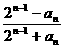等于(　　 )

A.-1　　　　　 B.1　　　　 C.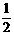D.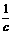an=an-1·c,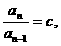=c,